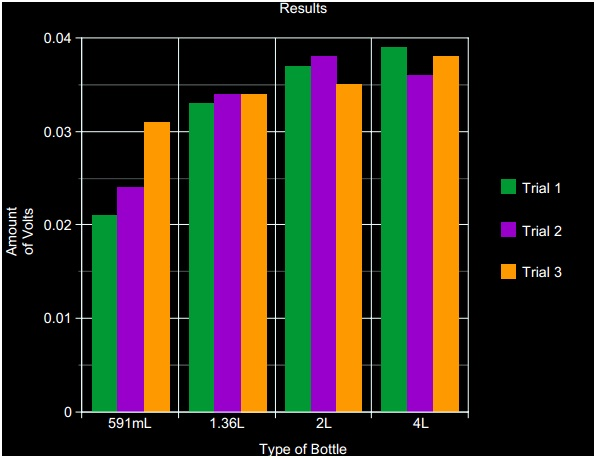Published on Mar 28, 2020

## Water Flow

### Abstract

The objective: Our project was to see if there is more water poured down will there be more electric current. Our hypothesis was if we used more water there would be more electric current. We first set up the pipe on an angle, and then we slowly poured the water down the pipe. We did 3 trials for each bottle (591mL, 1.36L, 2L, 4L). Then we looked at the multimeter then took the measurement in volts and typed it up. We found out that the 4L jug created more electric current. The 4L bottle gave out an average of 0.037 volts and our highest measurement was 0.039 volts.

### Purpose

Our problem is will the amount of water poured down the stream affect the amount of volts created?

### Hypothesis

If we use more water then there will be more volts created.

### Materials

* Small electric motor

* Pen

* Cardboard

* Tape

* Sticky Tack

* Tinfoil

* 1.36L bottle

* 591mL bottle

* 2L bottle

* 4L bottle

* Digital Multimeter

* Hot glue

* Scissors

* Pipe

* Popsicle Sticks

* Hot glue

* Paper towel

* Cement and Wood Bricks

### Procedure

1. Pour water from 591mL bottle.

2. Look at the multimeters reading.

3. Write down the volts.

4. Pour water from 1.36L bottle.

5. Look at the multimeters reading.

6. Write down the volts.

7. Pour water from 2L bottle.

9. Write down the volts.

10. Pour water from 4L bottle.

11. Look at the multimeters reading.

12. Write down the volts.

13. Look and see which amount of water reached the most amounts of volts.

### Observations

First we had the turbine in the pipe and that didn’t seem to work so we took the turbine and put it vertical outside of the pipe and it actually worked.Will the amount of water poured down the stream affect the amount of volts created? We thought if we use more water there will be more volts reached. We concluded that the 4L bottle created the most electric current and it was the largest bottle, so our hypothesis was correct. We did not deal with any safety. We would revise the experiment by getting a more variety of water bottles different sizes and using a different type of electric current measuring. We would then ask what water bottle gave the most electric current measured in both volts and amps. This could apply to the real world by using it on a larger scale; we could produce electricity for more places worldwide.

Our Independent variable was the amount of water we used to pour down the stream. Example: 4L bottle and 591mL bottle. Its role in the experiment was when we poured the water down the stream which created the electricity when it spun the turbine.

Our Dependent variable was the amount of volts created. Example: 0.039 volts. Its role in the experiment was to give us our results to see which amount of water created the most electricity.

Our Control Variables were the generator, the turbine, the length of the pipe, the speed of the water poured down, the electric current measurement. If we didn’t pour the water at about the same speed than if we pored it faster for one bottle and not the other it would have gave us false results. Also if we didn’t use the same turbine because one turbine might spin better than the other turbine it would have gave us false results.

### Conclusion

We had three trials for each bottle and we concluded that the more water we poured down, the more volts were created, so our hypothesis was correct.

### Bibliography

Websites we used were…

• http://www.technologystudent.com/energy1/watr1.htm

• http://en.wikipedia.org/wiki/Hydroelectricity

• http://water.usgs.gov/edu/hyhowworks.html

• http://education.nationalgeographic.com/education/encyclopedia/hydroelectric-energy/?ar_a=1